GRE Subject Test: Physics : Interference

Example Questions

Example Question #1 : Interference

A double slit experiment is set up with the following parameters: two slits are a separated by a distance. A beam of light with wavelength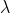shines through the two slits, and is projected onto a screen a distancefrom the slits. What is the distance on the screen between the central band and the next band on either side? (This distance is marked '' on the figure).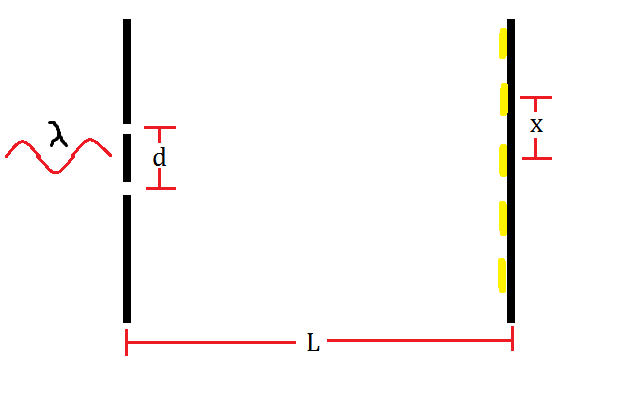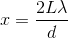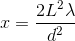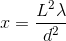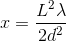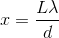Explanation:

The condition for constructive interference with double slit diffraction is given by: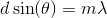Whereis 0, 1, 2, ...

Solving for the angle and using the small angle approximation, we get;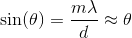The distancein the diagram can be related to the other quantities by simple geometry: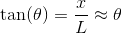Again, with the help of the small angle approximation. Setting the two thetas equal to each other and solving for, we get: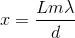For the central band,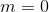, so theposition is also zero. The next band,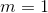, yields a distance of:Example Question #2 : Interference

Two waves with frequencies: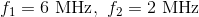are combined. What is the frequency of the resulting beat?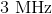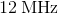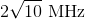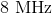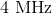Explanation:

The beat from two combined sound waves is: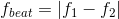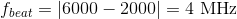All GRE Subject Test: Physics Resources